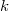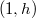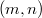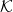﻿

###$k$-sets of type$(1, h)$ in finite planar spaces

#### Abstract

A set of type$(m,n)$ is a set$\mathcal K$ of points of a planarspace with the  property that each plane of the space meets$\mathcal K$ either in$m$ or$n$ points, and there are both planesintersecting$\mathcal K$ in$m$ points and in$n$ points. Inthis paper, sets of type$(1, h)$ in a planar space whose planespairwise intersect either in the empty--set or in a line, are studied.

DOI Code: 10.1285/i15900932v29n2p191

Keywords:
Linear spaces; projective planes; semiaffine planes; maximal arcs

Full Text: PDF